Next: Two-Dimensional Vortex Filaments Up: Two-Dimensional Incompressible Inviscid Flow Previous: Two-Dimensional Uniform Flow

# Two-Dimensional Sources and Sinks

Consider a uniform line source, coincident with the-axis, that emits fluid isotropically at the steady rate of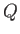unit volumes per unit length per unit time. By symmetry, we expect the associated steady flow pattern to be isotropic, and everywhere directed radially away from the source. (See Figure 5.3.) In other words, we expect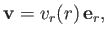(5.31)

where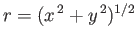. Consider a cylindrical surfaceof unit height (in the-direction) and radiusthat is co-axial with the source. In a steady state, the rate at which fluid crosses this surface must be equal to the rate at which the section of the source enclosed by the surface emits fluid. Hence,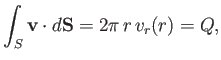(5.32)

which implies that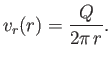(5.33)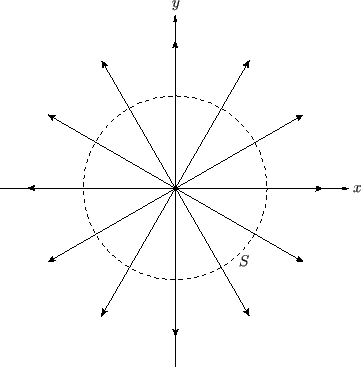According to Equations (5.13) and (5.14), the stream function associated with a line source of strengththat is coincident with the-axis is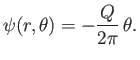(5.34)

Note that the streamlines,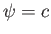, are directed radially away from the-axis, as illustrated in Figure 5.3. Note, also, that the stream function associated with a line source is multivalued. However, this does not cause any particular difficulty, because the stream function is continuous, and its gradient is single valued.

It follows from Equation (5.34) that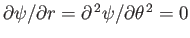. Hence, according to Equation (5.15),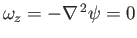. In other words, the steady flow pattern associated with a uniform line source is irrotational, and can, thus, be derived from a velocity potential. In fact, it is easily demonstrated that this potential takes the form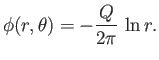(5.35)

A uniform line sink, coincident with the-axis, which absorbs fluid isotropically at the steady rate ofunit volumes per unit length per unit time has an associated steady flow pattern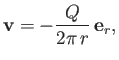(5.36)

whose stream function is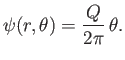(5.37)

This flow pattern is also irrotational, and can be derived from the velocity potential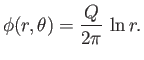(5.38)

Consider a line source and a line sink of equal strength, which both run parallel to the-axis, and are located a small distance apart in the-plane. Such an arrangement is known as a doublet or dipole line source. Suppose that the line source, which is of strength, is located at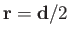(where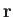is a position vector in the-plane), and that the line sink, which is also of strength, is located at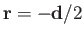. Let the function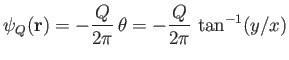(5.39)

be the stream function associated with a line source of strengthlocated at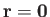. Thus,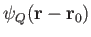is the stream function associated with a line source of strengthlocated at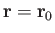. Furthermore, the stream function associated with a line sink of strengthlocated atis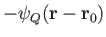. We expect the flow pattern associated with the combination of a source and a sink to be the vector sum of the flow patterns generated by the source and sink taken in isolation. It follows that the overall stream function is the sum of the stream functions generated by the source and the sink taken in isolation. In other words,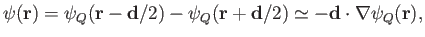(5.40)

to first order in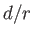. Hence, if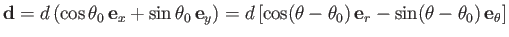, so that the line joining the sink to the source subtends a (counter-clockwise) angle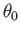with the-axis, then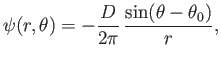(5.41)

where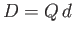is termed the strength of the dipole source. The previous stream function is antisymmetric across the line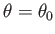joining the source to the sink. It follows that the associated dipole flow pattern,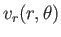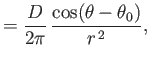(5.42)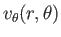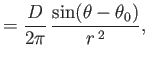(5.43)

is symmetric across this line. Figure 5.4 shows the streamlines associated with a dipole flow pattern characterized by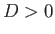and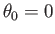. Note that the flow speed in a dipole pattern falls off like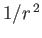.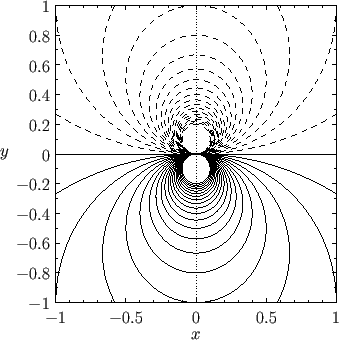A dipole flow pattern is necessarily irrotational because it is a linear superposition of two irrotational flow patterns. The associated velocity potential is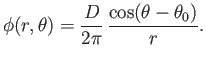(5.44)Next: Two-Dimensional Vortex Filaments Up: Two-Dimensional Incompressible Inviscid Flow Previous: Two-Dimensional Uniform Flow
Richard Fitzpatrick 2016-03-31